When I started learning Scheme, I took the common approach of learning a new language by implementing features from familiar languages (namely R). That approach sent me down the path of writing the `chez-stats` and `dataframe` libraries and porting `gnuplot-pipe` from Chicken to Chez Scheme. Those three libraries now allow me to conduct simple exploratory data analysis (EDA) in Chez Scheme that should be feel relatively familiar to R programmers. In this post, I will work through a simple example, which mostly serves to reinforce how much better suited R is for these types of tasks.

For this post, we will use the Texas housing data included as part of the `ggplot2` package for R. I've written that dataset to a CSV file for use in this post. First, let's import the necessary libraries (after following installation instructions in the repos linked above).

``````(import (chez-stats)
(dataframe)
(prefix (gnuplot-pipe) gp:))
``````

`chez-stats` has a procedure for reading delimited text files, `read-delim`, where the default separator is a comma.

``````> (list-head (read-delim "txhousing.csv") 5)
(("city" "year" "month" "sales" "volume" "median" "listings" "inventory" "date")
("Abilene" "2000" "1" "72" "5380000" "71400" "701" "6.3" "2000")
("Abilene" "2000" "2" "98" "6505000" "58700" "746" "6.6" "2000.0833333333333")
("Abilene" "2000" "3" "130" "9285000" "58100" "784" "6.8" "2000.1666666666667")
("Abilene" "2000" "4" "98" "9730000" "68600" "785" "6.9" "2000.25"))
``````

`read-delim` reads a CSV file as a list of lists with a row orientation. In the `dataframe` library, I refer to this type of list as a rowtable to distinguish it from the column-oriented dataframe, which is a record type based on an association list (alist).

The `dataframe` library includes pipeline operators taken from on an early implementation of SRFI 197 . The `->` places the output of the previous expression as the first argument of the next expression.

The `#t` in `rowtable->dataframe` indicates that the the rowtable has a header row. `read-delim` reads all data as strings. `dataframe-modify-at` allows us to map the same procedure, `string->number`, over several columns (e.g., `year`, `sales`, etc.).

``````> (define df1
(rowtable->dataframe #t)
(dataframe-modify-at
string->number 'year 'month 'sales 'volume 'median 'listings 'inventory 'date)))

> (dataframe-display df1)
dim: 8602 rows x 9 cols
city   year  month  sales    volume  median  listings  inventory       date
Abilene  2000.     1.    72.  5.380E+6  71400.      701.     6.3000  2000.0000
Abilene  2000.     2.    98.  6.505E+6  58700.      746.     6.6000  2000.0833
Abilene  2000.     3.   130.  9.285E+6  58100.      784.     6.8000  2000.1667
Abilene  2000.     4.    98.  9.730E+6  68600.      785.     6.9000  2000.2500
Abilene  2000.     5.   141.  1.059E+7  67300.      794.     6.8000  2000.3333
Abilene  2000.     6.   156.  1.391E+7  66900.      780.     6.6000  2000.4167
Abilene  2000.     7.   152.  1.264E+7  73500.      742.     6.2000  2000.5000
Abilene  2000.     8.   131.  1.071E+7  75000.      765.     6.4000  2000.5833
Abilene  2000.     9.   104.  7.615E+6  64500.      771.     6.5000  2000.6667
Abilene  2000.    10.   101.  7.040E+6  59300.      764.     6.6000  2000.7500
``````

In Chez Scheme, there is no built-in representation for missing values (like `NA` in R). We have to handle them manually. In our example, we have already converted 8 of the 9 columns from strings to numbers and can use `#f` as an indicator of missing values. But this carries the limitation that typos (e.g., `701t`) are treated as missing .

``````> (map string->number '("one" "2" ""))
(#f 2 #f)
``````

Here is a procedure for counting the number of `#f` values in each column of a dataframe. Because a dataframe record type is based on an alist, we can extract the alist from the dataframe with `dataframe-alist` and use standard Scheme procedures.

``````> (define (count-false df)
(map (lambda (col)
(length (filter (lambda (val) (not val)) (cdr col))))
(dataframe-alist df)))

> (count-false df1)
(0 0 0 568 568 616 1424 1467 0)
``````

We need a dataframe with no missing values because we can't pass missing values to the statistics and plotting procedures. Let's drop the columns with the most missing values and then drop all rows with any `#f` values. The filter expression relies on the fact that all values (other than `#f`) count as true in a Scheme boolean expression.

``````>  (define df-complete
(-> df1
(dataframe-drop 'listings 'inventory)
(dataframe-filter-all (lambda (x) x))))

> (count-false df-complete)
(0 0 0 0 0 0 0)

> (dataframe-display df-complete)
dim: 7985 rows x 7 cols
city   year  month  sales    volume  median       date
Abilene  2000.     1.    72.  5.380E+6  71400.  2000.0000
Abilene  2000.     2.    98.  6.505E+6  58700.  2000.0833
Abilene  2000.     3.   130.  9.285E+6  58100.  2000.1667
Abilene  2000.     4.    98.  9.730E+6  68600.  2000.2500
Abilene  2000.     5.   141.  1.059E+7  67300.  2000.3333
Abilene  2000.     6.   156.  1.391E+7  66900.  2000.4167
Abilene  2000.     7.   152.  1.264E+7  73500.  2000.5000
Abilene  2000.     8.   131.  1.071E+7  75000.  2000.5833
Abilene  2000.     9.   104.  7.615E+6  64500.  2000.6667
Abilene  2000.    10.   101.  7.040E+6  59300.  2000.7500
``````

Now that we have cleaned up the dataset we will aggregate the data for plotting to look for annual and seasonal patterns. `dataframe-aggregate` takes an `aggregate-expr` as one of its arguments. If we separately define an `aggregate-expr`, then we can easily use it with different grouping variables.

``````> (define agg-expr
(aggregate-expr
(avg-sales (sales) (exact->inexact (mean sales)))
(avg-volume (volume) (exact->inexact (mean volume)))
(avg-median (median) (exact->inexact (mean median)))))

> (define df-agg-year
(dataframe-aggregate df-complete '(year) agg-expr))

> (dataframe-display df-agg-year)
dim: 16 rows x 4 cols
year  avg-sales  avg-volume  avg-median
2000.   491.1244    7.379E+7    96442.00
2001.   510.4035    7.905E+7   100801.55
2002.   547.1171    8.820E+7   104365.11
2003.   540.1277    8.865E+7   108030.64
2004.   577.2337    9.738E+7   111096.75
2005.   635.9074    1.138E+8   118384.31
2006.   664.8815    1.247E+8   124263.48
2007.   620.6458    1.220E+8   130156.63
2008.   520.2976    1.019E+8   131297.93
2009.   470.6421    8.935E+7   131483.76
``````

We can plot data using `gnuplot-pipe`; a Chez Scheme interface for `Gnuplot`. I have only a rudimentary understanding of how to use `Gnuplot` so these plots will be very simple.

Below is an example of the `gnuplot-pipe` syntax for a simple line plot. The legend label is followed by lists for the x- and y-values.

``````(gp:call/gnuplot
(gp:plot "title 'x^2'" '(1 2 3 4) '(1 4 9 16))
(gp:save "OneLine.png"))
``````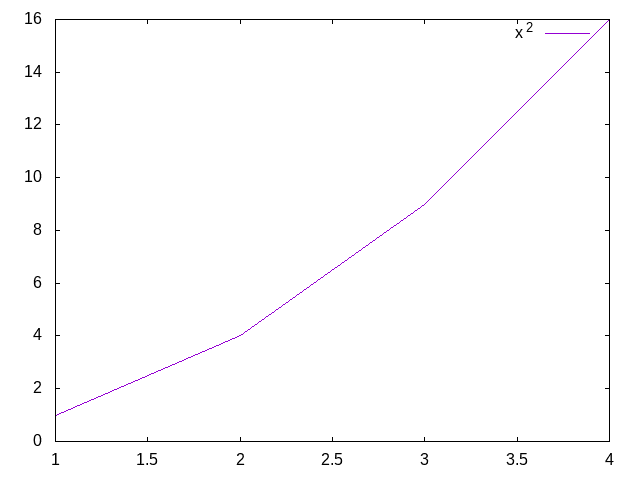When you want to plot multiple lines, you put the same syntax from the single line plot into a list with each element of the list representing a different line.

``````(gp:call/gnuplot
(gp:plot '(("title 'x^2'" (1 2 3 4) (1 4 9 16))
("title 'x^3'" (1 2 3 4) (1 8 27 64))))
(gp:save "TwoLines.png"))
``````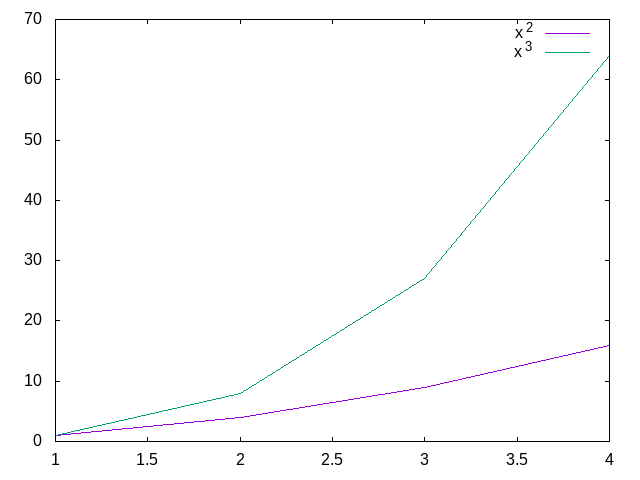The code for a plot with multiple lines based on a dataframe would be verbose and redundant. We can write a macro to simplify the plotting code. The `plot-expr` macro takes a dataframe as the 1st argument, x and y column names as the 2nd and 3rd arguments, and the grouping column name as an optional 4th argument. When a grouping argument is specified, `plot-expr` maps over the unique values in the grouping column to create the list structure expected by `gp:plot`.

``````(define-syntax plot-expr
(syntax-rules ()
[(_ df x y)
(list (list "" (\$ df (quote x)) (\$ df (quote y))))]
[(_ df x y grp)
(map (lambda (grp-val)
(let ([df-sub (dataframe-filter df (filter-expr (grp) (string=? grp grp-val)))])
(list
(string-append "title '" grp-val "'")
(\$ df-sub (quote x))
(\$ df-sub (quote y)))))
(dataframe-values-unique df (quote grp)))]))
``````

We can now use our `plot-expr` to plot the data that we aggregated by year across all cities and months. Here we are plotting the average median sale price by year. Nationally, home prices peaked in 2006 and bottomed out in 2012 (source), but, in Texas, housing prices mostly stayed flat during that period.

``````(gp:call/gnuplot
(gp:send "unset key")          ; remove legend
(gp:plot (plot-expr df-agg-year year avg-median))
(gp:save "MedianSalePriceByYear.png"))
``````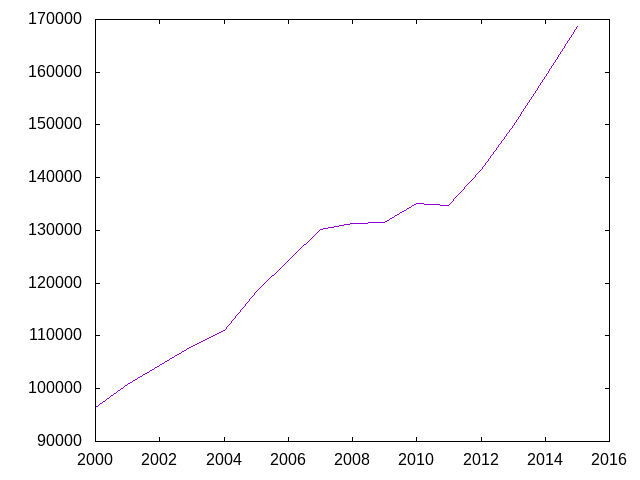Next, we create a `filter-expr` to filter by the largest cities in future aggregations.

``````(define city-filter
(filter-expr
(city)
(member city '("Austin" "Dallas" "El Paso" "Houston" "Lubbock" "San Antonio"))))
``````

In this next code block, we filter for the selected cities, aggregate by city and year, and then bind the previously aggregated dataframe, `df-agg-year`, while adding a city column to `df-agg-year` with the value of `All` in every row.

``````(define df-agg-city-year
(->  df-complete
(dataframe-filter city-filter)
(dataframe-aggregate '(city year) agg-expr)
(dataframe-bind
(dataframe-modify
df-agg-year
(modify-expr (city () "All"))))))
``````

The next plot includes 7 lines, but the `plot-expr` is mostly the same as for plotting one line.

``````(gp:call/gnuplot
(gp:send "set key top left")
(gp:plot (plot-expr df-agg-city-year year avg-median city))
(gp:save "MedianSalePriceByYearAndCity.png"))
``````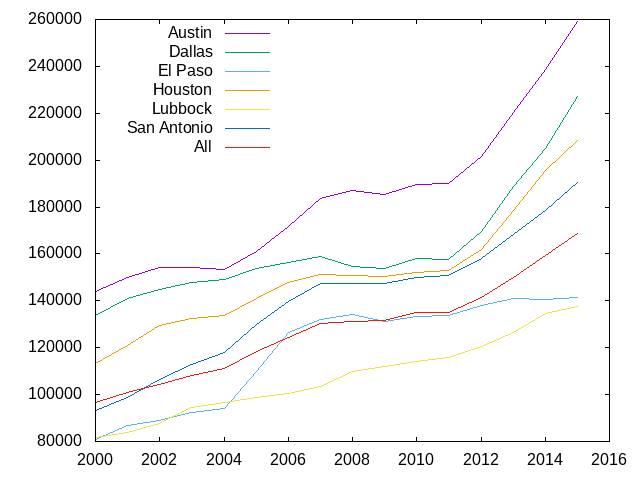The code is very similar for monthly patterns.

``````(define df-agg-month
(-> df-complete
(dataframe-aggregate '(month) agg-expr)))
(dataframe-sort (sort-expr (< month)))))

(define df-agg-city-month
(->  df-complete
(dataframe-filter city-filter)
(dataframe-aggregate '(city month) agg-expr)
(dataframe-sort (sort-expr (< month)))
(dataframe-bind
(dataframe-modify
df-agg-month
(modify-expr (city () "All"))))))

(gp:call/gnuplot
(gp:send "set key top left")
(gp:plot (plot-expr df-agg-city-month month avg-median city))
(gp:save "MedianSalePriceByMonthAndCity.png"))
``````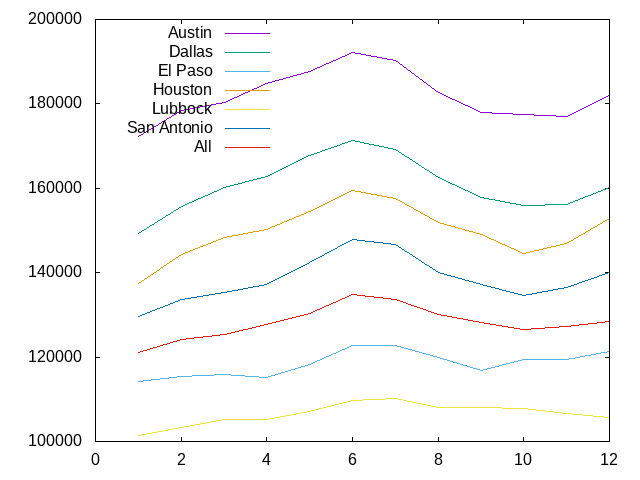In conclusion, it's been fun to see that something I built is moderately useful for exploratory data analysis, but it would be a HUGE amount of work to take these libraries to a place where it could even partially replace R for me. To be clear, I wasn't trying to replace R. I was just learning Scheme. I don't anticipate investing too much more time in the `dataframe` or `gnuplot-pipe` libraries, but I might slowly add features to `chez-stats`.

 In retrospect, `read-delim` is a better fit for the `dataframe` library.

 SRFI 197 has now deviated considerably from that early syntax.

 I'm not suggesting that this is an insurmountable problem, but it requires extra processing that is not done in this example.